# Copy Paste vs Fill Handle Copy with Tables References in an Excel formula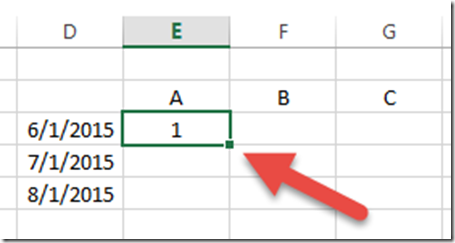In this recent post:

Friday Challenge Answers – Cumulative Events Over Time

I created a formula that referenced a table.  As I created my video I noticed a problem.  My Excel formula wouldn’t copy correctly to additional columns.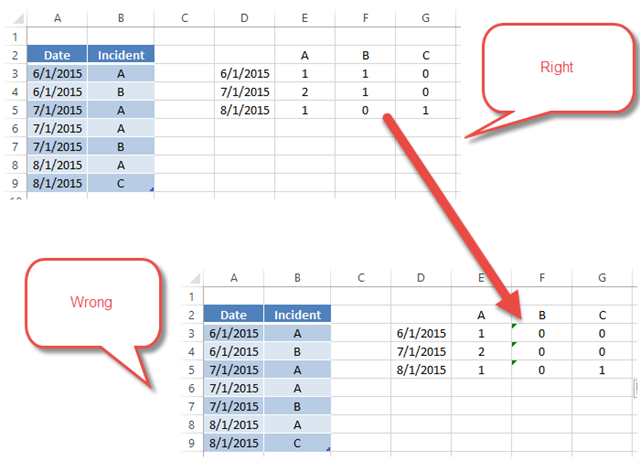But it worked when I created it and then as I was making my video it wasn’t working.  I was going crazy.

Then I figured it out!  It wasn’t working when I used the Fill Handle to copy/paste the formula across columns.But if I used CRTL+C copy and CTRL+V to paste across my range, it worked fine.  What is the deal?

The issue is that the Fill Handle treats table references as a Relative Reference.  Copy/Paste or CTRL+C & CTRL+V treats table references as an Absolute Reference or at least it doesn’t modify the formula when you copy and paste it across columns.

Here is my formula in Column E:

=COUNTIFS(Table1[Date],\$D3,Table1[Incident],E\$2)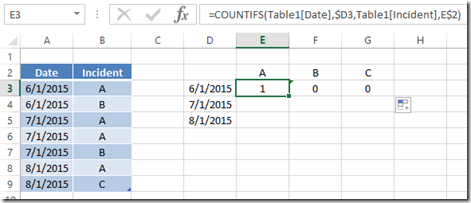If I use the Fill Handle and copy it to Column F, here is how the formula looks:

=COUNTIFS(Table1[Incident],\$D3,Table1[Date],F\$2)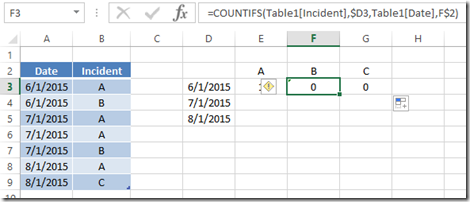Excel has shifted the formula table references by one column.  It does the same in column G, but that ends up working as Excel shifts it back to the first column after it hits the end of the table columns.  Here are a few ways to fix this if it is a problem for your formulas.

How to stop Excel from treating table formula references as relative and act more like absolute references:

# If you found the website and tutorials helpful, please consider donating to keep the lights on.

## Donate with PayPal here:1) Fix Version 1 – Use Copy/Paste Instead of the Fill Handler

If you just use Copy and Paste without using the Fill Handle, here is what column F looks like:

=COUNTIFS(Table1[Date],\$D3,Table1[Incident],F\$2)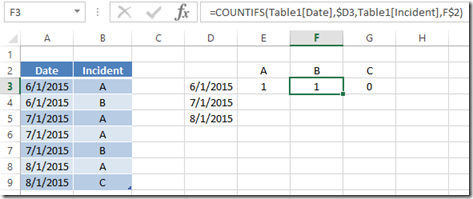Excel shifts the regular cell references as expected and it keeps the table references as though they were absolute references.  Copy/paste is one way to go.  There is another way to also fix this possibly unwanted outcome.

2) Fix Version 2 – Create Absolute Table References in Your Formula.

You can actually change your formula that references tables to act as an Absolute Reference instead of the Relative References that we were seeing in our sample formula.

You will need to change your original formula from this:

=COUNTIFS(Table1[Date],\$D3,Table1[Incident],E\$2)

To This:

=COUNTIFS(Table1[[Date]:[Date]],\$D3,Table1[[Incident]:[Incident]],E\$2)

To make your formula references to a table absolute, you will need to put an additional [ ] wrapper around a double column range reference

i.e. From this:    Table1[Date]    to this     Table1[[Date]:[Date]]

If you do this, you can THEN use the Fill Handle to copy and paste the formula and get the formula that we wanted.  Here is what the formula looks like when I copy and paste it using the fill handle from an adjacent column.

=COUNTIFS(Table1[[Date]:[Date]],\$D3,Table1[[Incident]:[Incident]],F\$2)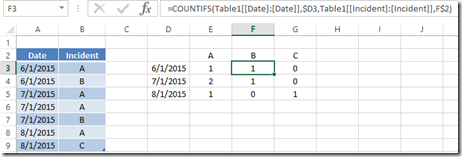Problem solved.  Now I know and knowing is half the battle 🙂

Here is a short video demonstrating the problems that I encountered and showing you how to fix it.

I don’t use Excel Tables enough in my work, but I am starting to do it more and more.  Tables are powerful in Excel, but when you use them in conjunction with formulas, you will want to remember which copy/paste technique that that will work best.  I think I will NOT use the handle in most cases.  This didn’t work as I expected using the fill handle.  What do you think?  Let me know in the comments below if you have run into this before.

Steve=True

1.Ken G

In this instance the use of Tables creates challenges. This example raises a couple questions I have had for a while.

What are the advantages of putting data into a Table?

When should you use a Table verse just entering data into the spreadsheet?

•SteveEqualsTrue

Thanks Ken. Great comments and questions. Peter R. uses tables a lot in his work. I don’t do them as much.

One of the best use cases I have found I created this posting for:

How-to Make Dynamic Excel Dashboard Charts Using Tables
https://www.exceldashboardtemplates.com/how-to-make-dynamic-excel-dashboard-charts-using-tables/

Steve=True

•Pete

Tables do a lot of things for you. When used properly, formulas in, or in reference to data in tables should be written in table syntax. This will give a giant benefit in having the formulas auto-expand to fit the data as a data set grows, much in the manner of dynamic ranges, but without the hassle of creating them, or the volatility usually inherent in them.
When you write a formula in cell in a column of a table, the formula will automatically get populated in all of the other cells in that column, and as the data grows, they will automatically be in the new rows.
I think one of the most overlooked benefit of using tables and table syntax is that the formulas (once written) are much easier to understand and trace for someone else who is working with your file. It is much easier to understand Table1[@Cost]*Table1[@Fee] is what makes up your price column as opposed to \$C32*\$F32. You don’t have to hunt for what is in columns C and F and then find the headers of those columns to understand what is happening.

There are many other benefits to tables. A good resource for tables is the book “Excel Tables: A Complete Guide” by Zack Barresse and Kevin Jones.

I hope that helps.

-Pete

•SteveEqualsTrue

•Pete

Also, if you have formulas written in table syntax such a VLOOKUP (or any other formula), you can rearrange the data in the a table and the formulas still work. However, if you work with a normal range and rearrange data, or add/delete columns, many formulas will break. A classic example is if you insert a column into a VLOOKUPs lookup table, the formula will break. If you use tables and table syntax, things are much more flexible. And in the specific case of VLOOKUP, an INDEX/MATCH combination along with table syntax is extremely flexible and data sets can easily be modified.
-Pete

•SteveEqualsTrue

Pete, another great example of the usefulness of tables. Thanks much!

•Ken G

Pete,
Thank you. I am going to have to do some learning. None of the info I have looked at so far through Excel Help led me to “table syntax”. I have started using tables in a few instances for the benefit of formulae automatically copying down.
Ken